In :
using PyPlot
using LinearAlgebra
using Statistics
using Random
import Base.MathConstants.e


# Part 1: Hyperparameter Optimization¶

Let's look at using stochastic gradient descent with various methods to optimize logistic regression. First, we'll generate a training set at random from the generative model associated with logistic regression. This generative model is, for label $y \in \{-1,1\}$, features $x \in \mathbb{R}^d$ and model $w \in \mathbb{R}^d$,

$$\mathbf{P}_w(y | x) = \frac{1}{1 + \exp(-y x^T w)}.$$

This means that if we make a bunch of independent observations, the total probability is

$$p(w) = \prod_{i=1}^N \frac{1}{1 + \exp(-y_i x_i^T w)}$$

and so maximizing this is equivalent to maximizing the log likelihood

$$\log p(w) = -\sum_{i=1}^N \log \left( 1 + \exp(-y_i x_i^T w) \right).$$

$$\nabla \log p(w) = -\sum_{i=1}^N \frac{\exp(-y_i x_i^T w) \cdot (-y_i x_i)}{1 + \exp(-y_i x_i^T w)}$$

which reduces to

$$\nabla \log p(w) = \sum_{i=1}^N \frac{y_i x_i}{1 + \exp(y_i x_i^T w)}.$$

Anyway, we can see that this corresponds to logistic regression.

In :
# generate the data
Random.seed!(424242)
d = 20;
N = 10000;
wtrue = randn(d);
wtrue = d^2 * wtrue / norm(wtrue);
X = randn(N, d);
X ./= sqrt.(sum(X.^2; dims=2));
Y = (1 ./ (1 .+ exp.(-X * wtrue)) .>= rand(N)) .* 2 .- 1;
sigma = 0.001;


Let's do logistic regression with regularization here. Our objective samples will be of the form

$$f_i(w) = -\log \left( 1 + \exp(-y_i x_i^T w) \right) + \frac{\sigma}{2} \| w \|^2$$

and the SGD updates will look like

$$w_{t+1} = w_t + \alpha_t \left( \frac{y_i x_i}{1 + \exp(y_i x_i^T w_t)} - \sigma w_t \right).$$

Let's look at the constants of strong convexity and Lipschitz continuity for this problem, to get a handle on the theory/optimal parameters. If we differentiate the objective twice, we get

$$\nabla^2 f_i(w) = x_i x_i^T \frac{1}{(1 + \exp(y_i x_i^T w_t)) (1 + \exp(-y_i x_i^T w_t))} + \sigma I.$$

It's pretty easy to see that

$$0 < \frac{1}{(1 + \exp(u)) (1 + \exp(-u))} \le \frac{1}{4},$$

and so since we initialized such that $\| x_i \|^2 = 1$, from the way we generated the examples, we can approximate

$$\sigma I \preceq \nabla^2 f_i(w) \preceq \left(\sigma + \frac{1}{4} \right) I.$$

So we can set $\mu = \sigma$ and $L = \sigma + \frac{1}{4}$. What about bounding the variance of the gradient samples? (Again here I'm using the nonstandard definition of variance for vectors: $\mathbf{Var}(X) = \mathbf{E}[\| X \|^2] - \| \mathbf{E}[ X ] \|^2$.) Well,

\begin{align*} \mathbf{Var}(\nabla f_i(w)) &= \mathbf{Var}\left( \frac{y_i x_i}{1 + \exp(y_i x_i^T w)} - \sigma w \right) \\ &= \mathbf{Var}\left( \frac{y_i x_i}{1 + \exp(y_i x_i^T w)} \right) \\ &\le \mathbf{E}\left[ \left\| \frac{y_i x_i}{1 + \exp(y_i x_i^T w)} \right\|^2 \right] \\ &\le \mathbf{E}\left[ \left\| x_i \right\|^2 \right] \\ &\le 1 \end{align*}

where this last line happens because we sampled $x_i$ uniformly from the unit ball. So we can set $M = 1$.

In :
mu = sigma;
L = sigma + 0.25;
M = 1;


What is the optimal step size for SGD under these conditions? Well, from Lecture 2, we had $$\alpha_t = \frac{2 \mu \| w_0 - w^* \|^2}{4 M + \mu^2 \| w_0 - w^* \|^2 t}$$ or $$\alpha_t = \frac{\alpha_0}{1 + \gamma t}$$ where $$\alpha_0 = \frac{2 \mu \| w_0 - w^* \|^2}{4 M}$$ and $$\gamma = \frac{\mu^2 \| w_0 - w^* \|^2}{4 M}.$$

In :
w0 = randn(d);

In :
function sgd_logreg(w0, alpha0, gamma, X, Y, sigma, niters, wopt)
w = w0
(N, d) = size(X)
dist_to_optimum = zeros(niters)
for k = 1:niters
alpha = alpha0 / (1 + gamma * (k-1));
i = rand(1:N)
xi = X[i,:];
yi = Y[i];
w = (1 - alpha * sigma) * w + alpha * xi * yi / (1 .+ exp.(yi * dot(xi, w)));
dist_to_optimum[k] = norm(w - wopt);
end
return (w, dist_to_optimum);
end

Out:
sgd_logreg (generic function with 1 method)
In :
# find the true minimum
function newton_logreg(w0, X, Y, sigma, niters)
N = size(X, 1);
d = size(X, 2);
w = w0;
for k = 1:niters
g = -X' * (Y ./ (1 .+ exp.(Y .* (X * w)))) + N * sigma * w;
H = X' * ((1 ./ ((1 .+ exp.(Y .* (X * w))) .* (1 .+ exp.(-Y .* (X * w))))) .* X) + N * sigma * I;
w = w - H \ g;
println("gradient norm: $(norm(g))") end return w end  Out: newton_logreg (generic function with 1 method) In : wopt = newton_logreg(wtrue, X, Y, sigma, 10);  gradient norm: 4000.0143011621494 gradient norm: 897.3056519964077 gradient norm: 233.1829505201235 gradient norm: 59.11585397232122 gradient norm: 5.912513207989782 gradient norm: 0.06801933640344747 gradient norm: 9.136508292096821e-6 gradient norm: 1.7241302462688477e-13 gradient norm: 5.164864426886177e-14 gradient norm: 5.6426423075409946e-14  In : alpha0 = 2 * mu * norm(w0 - wopt)^2 / (4 * M); gamma = mu^2 * norm(w0 - wopt)^2 / (4 * M);  In : Random.seed!(123456); (w, dto) = sgd_logreg(w0, alpha0, gamma, X, Y, sigma, 50000, wopt);  In : plot(dto) xlabel("iteration"); ylabel("distance to optimum");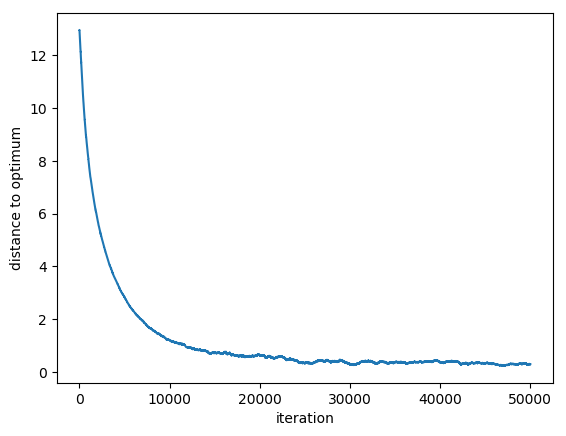Now let's try some different values of alpha and gamma. In : Random.seed!(123456); (w2, dto2) = sgd_logreg(w0, 2*alpha0, 4*gamma, X, Y, sigma, 50000, wopt); Random.seed!(123456); (w2, dto3) = sgd_logreg(w0, 3*alpha0, 9*gamma, X, Y, sigma, 50000, wopt);  In : semilogy(dto; label = "optimal step size") semilogy(dto2; label = "2x optimal") semilogy(dto3; label = "3x optimal") xlabel("iteration"); ylabel("distance to optimum"); legend();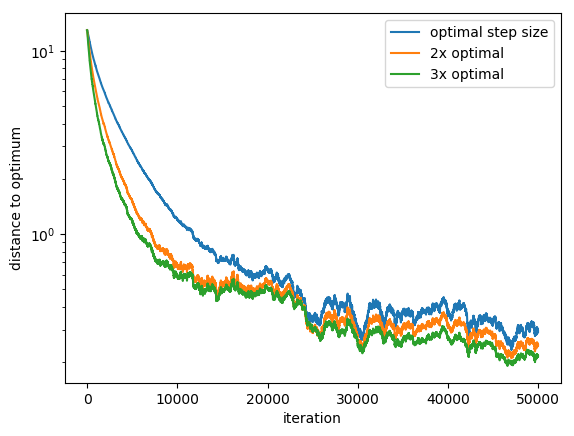What is the best assignment of the step size after 20000 iterations? In : ## do not re-run; takes too long etas = exp.(collect(-1:0.05:3)); dists = [ mean([sgd_logreg(w0, eta*alpha0, eta^2*gamma, X, Y, sigma, 20000, wopt)[end] for i=1:100]) for eta in etas ];  In : loglog(etas, dists); scatter(etas, dists); imin = argmin(dists); scatter(etas[imin], dists[imin]; color="red"); xlabel("step size scaled by"); ylabel("distance to optimum after 20000 steps");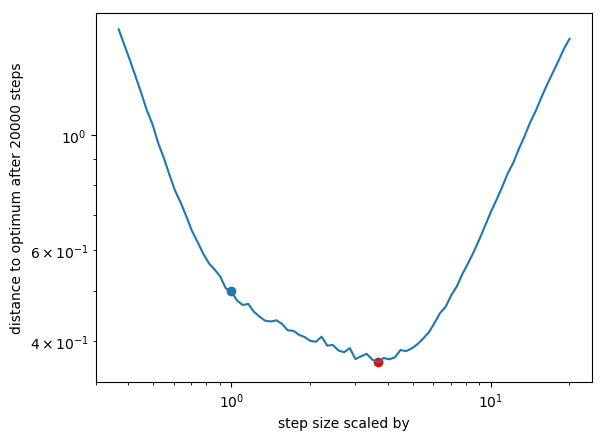Takeaway: the theory gave us something good, but not the best. What about other algorithms? Maybe if we ran SVRG, the theoretically optimal parameters would be correct. In : function svrg(w0, alpha, X, Y, sigma, niters, nepochs, wopt) w = w0 (N, d) = size(X) dist_to_optimum = zeros(niters * nepochs) for epi = 1:nepochs wtilde = w; gtilde = X' * (Y ./ (1 .+ exp.(Y .* (X * wtilde)))) / N - sigma * wtilde; for k = 1:niters i = rand(1:N) xi = X[i,:]; yi = Y[i]; w = w + alpha * (xi * yi / (1 .+ exp.(yi * dot(xi, w))) - sigma * w - xi * yi / (1 .+ exp.(yi * dot(xi, wtilde))) + sigma * wtilde + gtilde); dist_to_optimum[k + (epi-1)*niters] = norm(w - wopt); end end return (w, dist_to_optimum); end  Out: svrg (generic function with 1 method) Optimal step size from analysis in SVRG paper (assuming contraction factor of$e^{-1}\$ at each outer epoch) is $$\alpha = \frac{1}{4 L (e+1)}$$ and optimal epoch length was $$T = \frac{8 L e (e + 1)}{\mu}.$$

In :
alpha = 1 / (4 * L * (e+1));
T = Int64(ceil(8 * L * e * (e+1) / mu));
K = 10;

In :
time1 = @timed (w, dto_svrg) = svrg(w0, alpha, X, Y, sigma, T, K, wopt);

In :
semilogy(dto_svrg);
xlabel("iterations");
ylabel("distance to optimum");
title("Convergence of SVRG with Standard Parameters");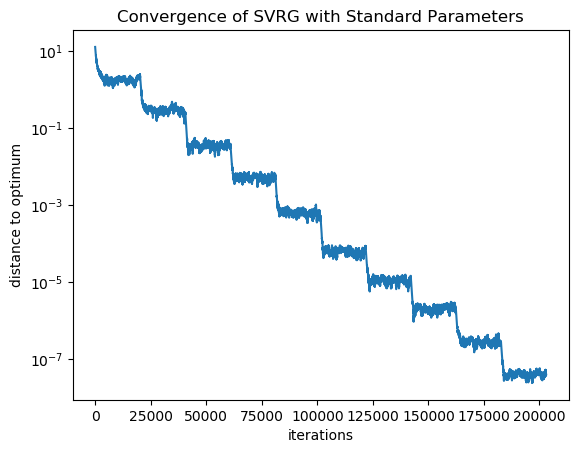Let's see what happens when we try a smaller step size.

In :
time2 = @timed (w2, dto_svrg2) = svrg(w0, alpha / 5, X, Y, sigma, Int64(ceil(T)), K, wopt);

In :
semilogy(dto_svrg; label = "optimal", color = "blue");
semilogy(dto_svrg2; label = "20% step size", color = "red");
xlabel("inner iteration")
ylabel("distance to optimum")
legend();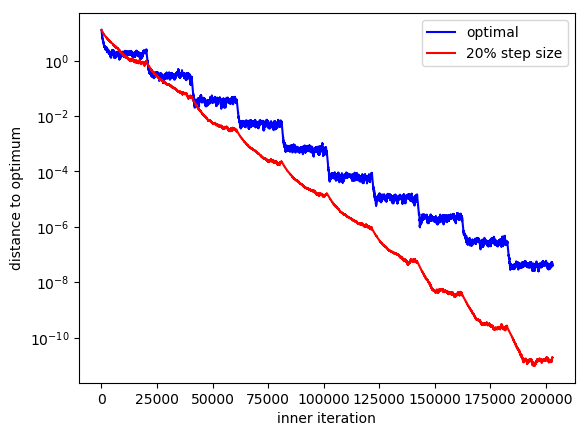In :
semilogy(collect(1:length(dto_svrg)) / length(dto_svrg) * time1, dto_svrg; label = "optimal", color = "blue");
semilogy(collect(1:length(dto_svrg2)) / length(dto_svrg2) * time2, dto_svrg2; label = "3x step size", color = "red");
xlabel("wall clock time (seconds)")
ylabel("distance to optimum")
legend();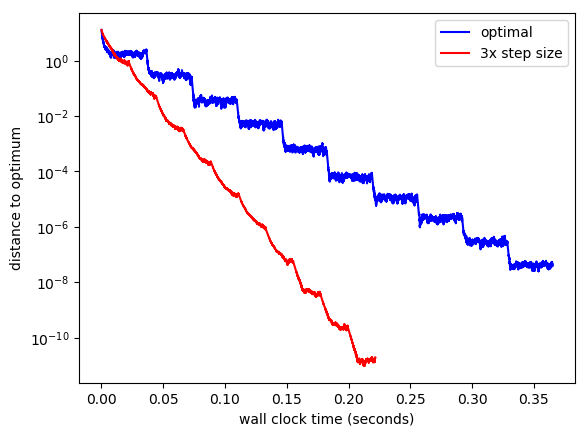Now, what if we also adjust the epoch length to be smaller?

In :
Random.seed!(123456);
time3 = @timed (w3, dto_svrg3) = svrg(w0, alpha / 2, X, Y, sigma, Int64(ceil(T / 2)), K, wopt);

In :
semilogy(dto_svrg; label = "optimal", color = "blue");
semilogy(dto_svrg2; label = "20% step size", color = "red");
semilogy(dto_svrg3; label = "50% epoch, 50% step size", color = "green");
xlabel("inner iteration")
ylabel("distance to optimum")
legend();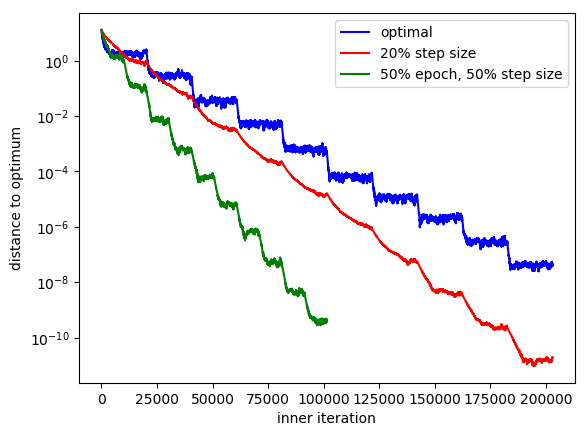In :
semilogy(collect(1:length(dto_svrg)) / length(dto_svrg) * time1, dto_svrg; label = "optimal", color = "blue");
semilogy(collect(1:length(dto_svrg2)) / length(dto_svrg2) * time2, dto_svrg2; label = "20% step size", color = "red");
semilogy(collect(1:length(dto_svrg3)) / length(dto_svrg3) * time3, dto_svrg3; label = "50% epoch, 50% step size", color = "green");
xlabel("wall clock time (seconds)")
ylabel("distance to optimum")
legend();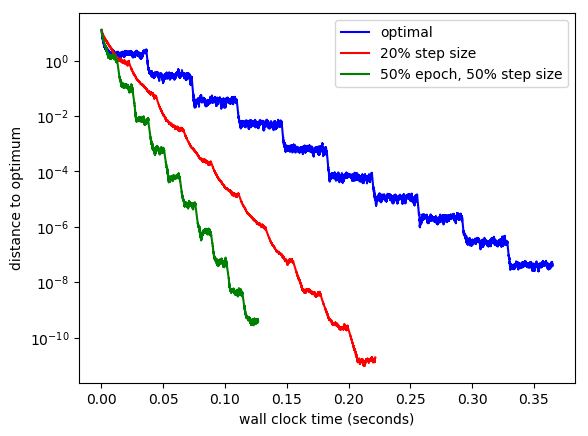.

.

.

.

.

.

.

.

.

.

.

.

.

.

.

.

.

.

.

.

.

.

.

.

.

.

.

.

.

.

.

.

.

.

.

# Part 2: Can you do better?¶

In :
alpha_class = 0.75*alpha;
T_class = Int64(ceil(T / 4));
K_class = Int64(ceil(2*K));

Random.seed!(123456);
time_class = @timed (w_class, dto_svrg_class) = svrg(w0, alpha_class, X, Y, sigma, T_class, K_class, wopt);

semilogy(collect(1:length(dto_svrg)) / length(dto_svrg) * time1, dto_svrg; label = "theoretical baseline", color = "blue");
semilogy(collect(1:length(dto_svrg3)) / length(dto_svrg3) * time3, dto_svrg3; label = "starting point", color = "green");
semilogy(collect(1:length(dto_svrg_class)) / length(dto_svrg_class) * time_class, dto_svrg_class; label = "your suggestion", color = "purple");
xlabel("wall clock time (seconds)")
ylabel("distance to optimum")
legend();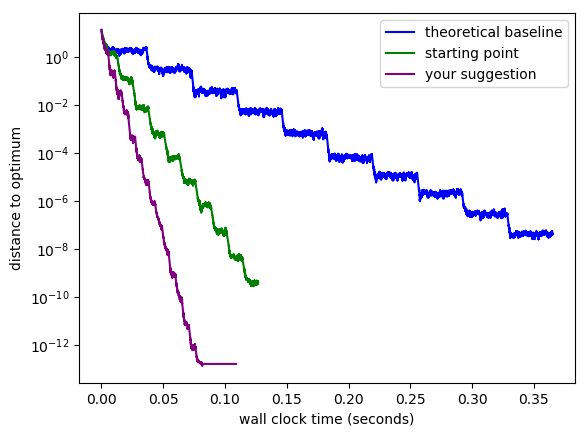In [ ]:


In [ ]:


In [ ]:


In :
using Iterators

ArgumentError: Package Iterators not found in current path:
- Run import Pkg; Pkg.add("Iterators") to install the Iterators package.

Stacktrace:
 top-level scope at In:1
In :
collect(Iterators.repeated((4,7),6))

Out:
6-element Array{Tuple{Int64,Int64},1}:
(4, 7)
(4, 7)
(4, 7)
(4, 7)
(4, 7)
(4, 7)
In [ ]:


In [ ]:


In [ ]:


In [ ]:


In :